# 我的Go+语言初体验——Go+语言构建神经网络实战手写数字识别

“我的Go+语言初体验” | 征文活动进行中......

# 1. 神经网络相关概念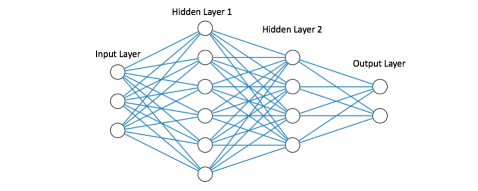虽然已经有一些现有的神经网络框架可以使用，但作为体验作，本文将从头开始构建简单的全连接网络，以更好了解神经网络的基本组成以及运行原理。

# 2. 构建神经网络实战手写数字识别

## 2.1 构建神经网络

### 2.1.1 节点计算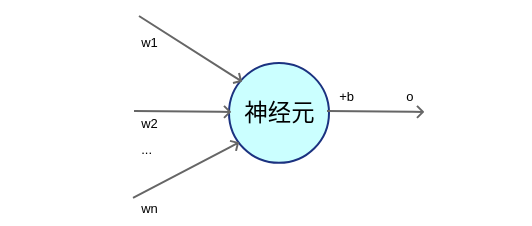使用公式进行描述，如下所示：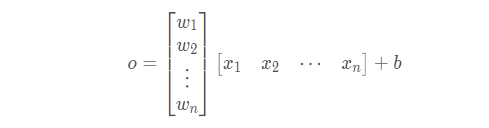``````    hiddenLayerInput.Mul(x, nn.wHidden)
addBHidden := func(_, col int, v float64) float64 { return v + nn.bHidden.At(0, col) }

gonum 是用于高效编写数字和科学算法的算法库，可以通过执行以下命令获取：

``go get gonum.org/v1/gonum``

### 2.1.2 激活函数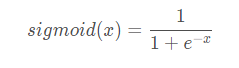在 Go+ 中实现 sigmoid 函数如下：

``````// 激活函数
func sigmoid(x float64) float64 {
return 1.0 / (1.0 + math.Exp(-x))
}``````

### 2.1.3 网络架构

``````config := neuralNetConfig{
// 输入层神经元
inputNeurons:  784,
// 输出层神经元
outputNeurons: 10,
// 隐藏层神经元
hiddenNeurons: 128,
// 训练 Epoch 数
numEpochs:     5000,
// 学习率
learningRate:  0.01,
}``````

## 2.2 读取手写数字MNIST数据集

1. 首先需要下载数据
2. 然后读取数据；

``````//读取数据
f, err := os.Open("new_mnist_train.csv")
if err != nil {
log.Fatal(err)
}
defer f.Close()

// 读取所有CSV记录
if err != nil {
log.Fatal(err)
}

// trainInputsData和trainLabelsData用于保存所有浮点值
trainInputsData := make([]float64, 784*len(mnistData))
println(len(inputsData))
trainLabelsData := make([]float64, 10*len(mnistData))

// 记录输入矩阵值的当前索引
var trainInputsIndex int
var trainLabelsIndex int

for idx, record := range mnistData {
// 跳过文件头
if idx == 0 {
continue
}

// 循环读取每行的每个数据
for i, val := range record {
// 将数据转换为浮点形
parsedVal, err := strconv.ParseFloat(val, 64)
if err != nil {
log.Fatal(err)
}

// 构造标签数据
if i == 0 || i == 1 || i == 2 || i == 3 || i == 4 || i == 5 || i == 6 || i == 7 || i == 8 || i == 9{
trainLabelsData[trainLabelsIndex] = parsedVal
trainLabelsIndex++
continue
}        // 构建输入数据
trainInputsData[trainInputsIndex] = parsedVal
trainInputsIndex++
}
}``````

3. 最后将数据整形，使得其加油可用于网络输入的形状。

``````inputs := mat.NewDense(len(mnistData), 784, trainInputsData)
labels := mat.NewDense(len(mnistData), 10, trainLabelsData)``````

## 2.3 训练神经网络

### 2.3.1 前向计算

``````    // 初始化网络权重和偏置值
wHiddenRaw := make([]float64, nn.config.hiddenNeurons*nn.config.inputNeurons)
bHiddenRaw := make([]float64, nn.config.hiddenNeurons)
wOutRaw := make([]float64, nn.config.outputNeurons*nn.config.hiddenNeurons)
bOutRaw := make([]float64, nn.config.outputNeurons)

for _, param := range [][]float64{wHiddenRaw, bHiddenRaw, wOutRaw, bOutRaw} {
for i := range param {
param[i] = randGen.Float64()
}
}    wHidden := mat.NewDense(nn.config.inputNeurons, nn.config.hiddenNeurons, wHiddenRaw)
bHidden := mat.NewDense(1, nn.config.hiddenNeurons, bHiddenRaw)
wOut := mat.NewDense(nn.config.hiddenNeurons, nn.config.outputNeurons, wOutRaw)
bOut := mat.NewDense(1, nn.config.outputNeurons, bOutRaw)``````

``````    // 前向计算过程
hiddenLayerInput := &mat.Dense{}
hiddenLayerInput.Mul(x, wHidden)
addBHidden := func(_, col int, v float64) float64 { return v + bHidden.At(0, col) }

hiddenLayerActivations := &mat.Dense{}
applySigmoid := func(_, _ int, v float64) float64 { return sigmoid(v) }
hiddenLayerActivations.Apply(applySigmoid, hiddenLayerInput)    outputLayerInput := &mat.Dense{}
outputLayerInput.Mul(hiddenLayerActivations, wOut)
addBOut := func(_, col int, v float64) float64 { return v + bOut.At(0, col) }
output.Apply(applySigmoid, outputLayerInput)``````

### 2.3.2 反向传播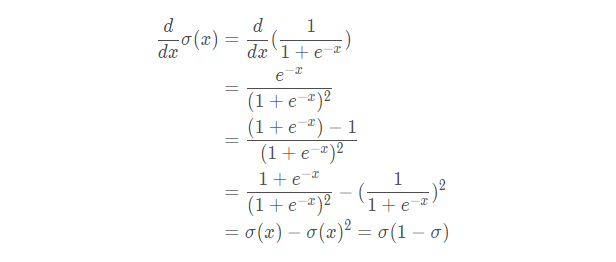使用  Go+ 语言实现代码如下:

``````func sigmoidDerivation(x float64) float64 {
return sigmoid(x) * (1.0 - sigmoid(x))
}``````

``````    //梯度的反向传播
networkError := &mat.Dense{}
networkError.Sub(y, output)    // 损失函数

slopeOutputLayer := &mat.Dense{}
applySigmoidDerivation := func(_, _ int, v float64) float64 { return sigmoidDerivation(v) }
slopeOutputLayer.Apply(applySigmoidDerivation, output)
slopeHiddenLayer := &mat.Dense{}
slopeHiddenLayer.Apply(applySigmoidDerivation, hiddenLayerActivations)

dOutput := &mat.Dense{}
dOutput.MulElem(networkError, slopeOutputLayer)
errorAtHiddenLayer := &mat.Dense{}
errorAtHiddenLayer.Mul(dOutput, wOut.T())

dHiddenLayer := &mat.Dense{}
dHiddenLayer.MulElem(errorAtHiddenLayer, slopeHiddenLayer)

// 参数修改

if err != nil {
return err
}

if err != nil {
return err
}

``````func sumAxis(axis int, m *mat.Dense) (*mat.Dense, error) {

numRows, numCols := m.Dims()
// println(numRows, numCols)

var output *mat.Dense

switch axis {
case 0:
result := make([]float64, numCols)
for i := 0; i < numCols; i++ {
col := mat.Col(nil, i, m)
result[i] = floats.Sum(col)
}
output = mat.NewDense(1, numCols, result)
case 1:
result := make([]float64, numRows)
for i := 0; i < numRows; i++ {
row := mat.Row(nil, i, m)
result[i] = floats.Sum(row)
}
output = mat.NewDense(numRows, 1, result)
default:
return nil, errors.New("invalid axis, must be 0 or 1")
}

return output, nil
}``````

## 2.4 评估神经网络

``````    // 使用经过训练的模型进行预测
predictions, err := network.predict(testInputs)
if err != nil {
log.Fatal(err)
}

// 计算模型预测准确率
var truePred int
numPreds, _ := predictions.Dims()
for i := 0; i < numPreds; i++ {

// 获取标签
labelRow := mat.Row(nil, i, testLabels)
var species int
for idx, label := range labelRow {
// println(idx, label)
if label == 1.0 {
// println(idx)
category = idx
break
}
}

// 计算预测正确的个数
if predictions.At(i, category) == floats.Max(mat.Row(nil, i, predictions)) {
// for j:= 0; j < 10; j++ {
//         println(j, mat.Row(nil, i, predictions)[j])
// }
truePred++
}
}

// 计算准确率
accuracy := float64(truePred) / float64(numPreds)

// 输出准确率
fmt.Printf("\nAccuracy = %0.2f\n\n", accuracy)``````

# 3. 程序运行

``gop run mnist_recognition.go``

``Accuracy = 0.89``

# 后记

...全文
692 16 打赏 收藏 举报

16 条回复

• 打赏
• 举报

@筑梦之行我辈义不容辞.
• 举报

• 打赏
• 举报

@清风明月晓我心 融会贯通，可以根据项目需要选择合适语言，嘿嘿，技多不压身.
• 举报

• 打赏
• 举报

@大数据分析师 这个博文主要是体验GO+，关于这个数学原理可以之后详细介绍，欢迎持续关注.
• 举报

• 打赏
• 举报

@头铁达人 多多通过编程联系吧，多用就能快速掌握.
• 举报

TechMaker 2021-12-09

• 打赏
• 举报

Go+ 2021-12-10
@TechMaker https://bbs.csdn.net/forums/goplus?typeId=19393 可以在这里了解、学习Go+语言哈
• 举报

@TechMaker 嗯，可以私信发我你邮箱，我给你发一份.
• 举报

@你的眼睛里有小星星 嘿嘿，共同学习.
• 举报

https://blog.csdn.net/LOVEmy134611/article/details/121757994

• 打赏
• 举报

768

Go+ 官方开发者社区。我们希望向广大的开发者和数据科学家介绍 Go+ 的定位和意义，并邀请更多开发者一起贡献代码、共建 Go+ 生态。 Go+ 官网：https://goplus.org/

Go+ 官网：https://goplus.org/
GitHub地址：https://github.com/goplus/gop